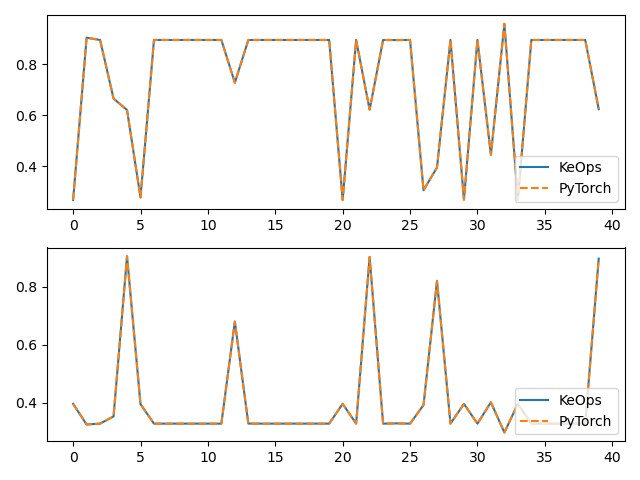# SumSoftMaxWeight reduction

Using the torch.Genred API, we show how to perform a computation specified through:

• Its inputs:

• $$x$$, an array of size $$M\times 3$$ made up of $$M$$ vectors in $$\mathbb R^3$$,

• $$y$$, an array of size $$N\times 3$$ made up of $$N$$ vectors in $$\mathbb R^3$$,

• $$b$$, an array of size $$N\times 2$$ made up of $$N$$ vectors in $$\mathbb R^2$$.

• Its output:

• $$c$$, an array of size $$M\times 2$$ made up of $$M$$ vectors in $$\mathbb R^2$$ such that

$c_i = \frac{\sum_j \exp(K(x_i,y_j))\,\cdot\,b_j }{\sum_j \exp(K(x_i,y_j))},$

with $$K(x_i,y_j) = \|x_i-y_j\|^2$$.

## Setup

Standard imports:

import time

import torch
from matplotlib import pyplot as plt

from pykeops.torch import Genred


Define our dataset:

M = 500  # Number of "i" points
N = 400  # Number of "j" points
D = 3  # Dimension of the ambient space
Dv = 2  # Dimension of the vectors

x = 2 * torch.randn(M, D)
y = 2 * torch.randn(N, D)
b = torch.rand(N, Dv)


## KeOps kernel

Create a new generic routine using the pykeops.numpy.Genred constructor:

formula = "SqDist(x,y)"
formula_weights = "b"
aliases = [
"x = Vi(" + str(D) + ")",  # First arg:  i-variable of size D
"y = Vj(" + str(D) + ")",  # Second arg: j-variable of size D
"b = Vj(" + str(Dv) + ")",
]  # Third arg:  j-variable of size Dv

softmax_op = Genred(
formula, aliases, reduction_op="SumSoftMaxWeight", axis=1, formula2=formula_weights
)

# Dummy first call to warmup the GPU and get accurate timings:
_ = softmax_op(x, y, b)


Use our new function on arbitrary Numpy arrays:

start = time.time()
c = softmax_op(x, y, b)
print("Timing (KeOps implementation): ", round(time.time() - start, 5), "s")

# compare with direct implementation
start = time.time()
cc = torch.sum((x[:, None, :] - y[None, :, :]) ** 2, 2)
cc -= torch.max(cc, dim=1)[:, None]  # subtract the max for robustness
cc = torch.exp(cc) @ b / torch.sum(torch.exp(cc), dim=1)[:, None]
print("Timing (PyTorch implementation): ", round(time.time() - start, 5), "s")

print("Relative error : ", (torch.norm(c - cc) / torch.norm(c)).item())

# Plot the results next to each other:
for i in range(Dv):
plt.subplot(Dv, 1, i + 1)
plt.plot(c.cpu().detach().numpy()[:40, i], "-", label="KeOps")
plt.plot(cc.cpu().detach().numpy()[:40, i], "--", label="PyTorch")
plt.legend(loc="lower right")
plt.tight_layout()
plt.show()Out:

Timing (KeOps implementation):  0.00021 s
Timing (PyTorch implementation):  0.00364 s
Relative error :  2.1595359100956557e-07


Total running time of the script: ( 0 minutes 0.133 seconds)

Gallery generated by Sphinx-Gallery